# Test: Basic Electronics- 3

## 20 Questions MCQ Test Mock Test Series for SSC JE Electrical Engineering | Test: Basic Electronics- 3

Description
Attempt Test: Basic Electronics- 3 | 20 questions in 12 minutes | Mock test for SSC preparation | Free important questions MCQ to study Mock Test Series for SSC JE Electrical Engineering for SSC Exam | Download free PDF with solutions
QUESTION: 1

### Generally, mobility of electrons in semiconductor is _________ times the mobility of holes:

Solution:

Mobility of electrons and holes depends on their effective masses. Effective mass of electrons is less than that of holes hence electrons have higher mobility than holes.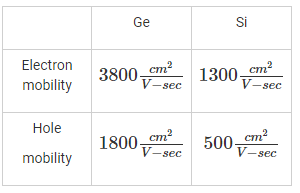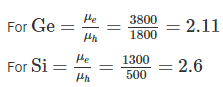For both Ge & Si μe > 2μh

Hence option (b) is correct.

QUESTION: 2

### In a p-n Junction with no externally applied voltage, the drift and diffusion components of the hole and electron currents:

Solution:

In a p-n Junction when no bias voltage is applied, the net current is zero.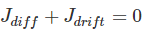Hence the drift and diffusion components of the hole and electron currents must sum to zero.

QUESTION: 3

### Fermi level is the measure of

Solution:

From the definition of fermi level, the fermi level is energy state having probability ‘1/2’ of being occupied by an electron.

QUESTION: 4

Semiconductor lasers are based on the following principle:

Solution:

LASER stands for Light Amplification by Stimulated Emission of Radiation. These are based on the principle of population inversion at a junction as explained in below figure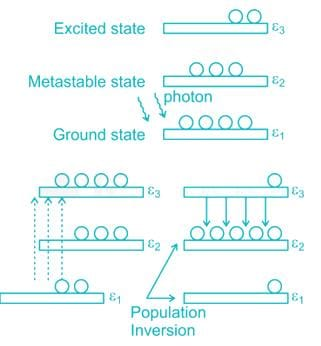QUESTION: 5

The circuit shown in the figure is a: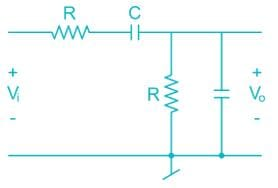Solution: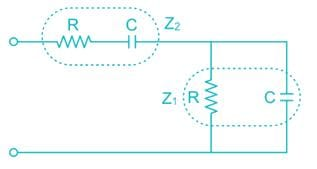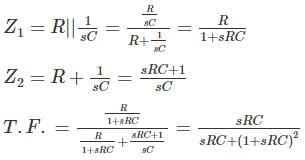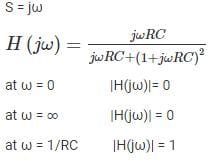The frequency Response is shown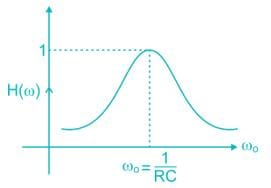Bandpass filters at ω0 = 1/RC

QUESTION: 6

The capacitance of a p-n junction diode:

Solution:

During forward Bias, the diffusion capacitance is the dominant capacitance.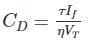τ = mean lifetime of minority carriers

If = forward current

As forward current Increase exponentially,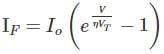The junction capacitance increases exponentially on forward bias.

QUESTION: 7

A light-emitting diode (LED) converts:

Solution:

LED works on the principle of electroluminescence which is emission of light under influence of electric field.

QUESTION: 8

A Bipolar Junction Transistor saturation point may be defined as

Solution:

For a BJT operating in active mode, the base current (Ib) and collector current (IC) are related as Ic = β Ib where β is the current gain.

At saturation the voltage across collector – emitter junction (VCE) is constant (∼ 0.2V) and collector current is independent of base current.

QUESTION: 9

In a rectifier, commutation of diodes refers to transferring energy from –

Solution:

Commutation in a rectifier is the process of transfer of current from one device (diode or thyristor) to the other in a rectifier. The device from which the current is transferred is called the outgoing device and the device to which the current is transferred is called the incoming device. The incoming device turns on at the beginning of commutation while the outgoing device turns off at the end of commutation.

Here, commutation of diodes refers to transferring energy from rectifier diode to freewheeling diode. Freewheeling diode is used to reduce the harmonics and also suppress the voltage spikes occur in inductive load.

QUESTION: 10

Which among the following has a frequency selective amplifier whose gain decreases from a finite value to zero as the frequency of the sinusoidal input increase form dc to infinity?

Solution:

The frequency response of LPF is shown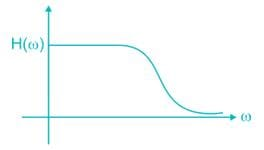From the frequency response it is clear that the gain of low pass filter decreases form finite value to zero as frequency is increased from 0 to ∞

QUESTION: 11

Consider the following circuit configurations:

1. Common emitter

2. Common base

3. Emitter follower

The correct sequence in increasing input impedance is

Solution: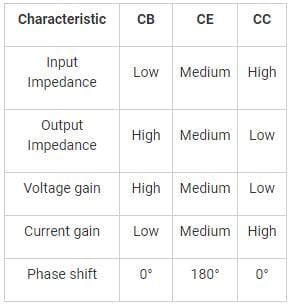QUESTION: 12

In a bipolar junction transistor, the current gain β.

Solution:

The current gain β is defined as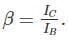At higher temperatures the thermal injection increases the collector current & hence β increases.

QUESTION: 13

The h-parameter equivalent circuit of a BJT is valid for:

Solution:

The h-parameters model doesn’t take into account junction capacitance which are significant at high frequencies. The h-parameter model is valid for signals with Vbe < 10 mV. Hence h-parameter model is suitable for low frequencies & small signals

QUESTION: 14

Transistors leakage current mainly depends on:

Solution:

The leakage current in a transistor mainly depends on temperature and doubles for every 10°C rise in temperature. This leakage current dependence on temperature is responsible for thermal run-away in transistors.

QUESTION: 15

The base region of a p – n – p transistor is:

Solution:

In pnp transistor, the base is n-type semiconductor i.e. it is doped with electrons. The width of the base region is kept small/thin to allow maximum carriers from emitter to pass through base to collector without much recombination

QUESTION: 16

A transistor is said to be in a quiescent stage when

Solution:

The Q-point in the Transistor is established for faithful Amplification in middle of DC-load line. The Q-point is established using DC voltage & resistance without any application of AC signal.

QUESTION: 17

In a BJT, collector region width is maximum when it.

1. Collects maximum number of charge carrier

2. Reduces heat dissipation per unit area Out of these

Solution:

The order of width is

wcollector > wemitter > wBase

The order of doping is

nemitter > ncollector > nbase

QUESTION: 18

DC bias is adjusted greater than its cut-off value so that the output current flow for less than half of the input voltage cycle, in:

Solution: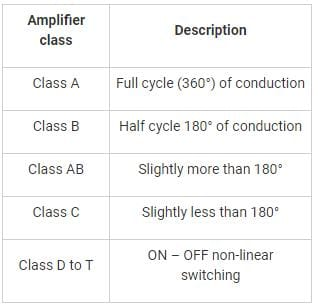QUESTION: 19

A tuned amplifier is used:

Solution:

Tuned amplifiers are employed for the purpose of selecting a particular frequency among set of available frequencies. Hence they are employed in radio receives.

QUESTION: 20

What is the required connection for an enhancement type N-MOSFET to function as a resistor?

Solution:

The overdrive voltage is defined as the voltage between transistor gate and source (VGS) in excess of the threshold voltage (VT) ie Vgs - Vt

For N-Mos to function as a resistor, it should be operated in linear region. I.e. VDS < Vgs – Vt

For using N-Mos as an amplifier, it should be operated in saturation region. I.e. VDS ≥ Vgs – VtUse Code STAYHOME200 and get INR 200 additional OFF Use Coupon Code